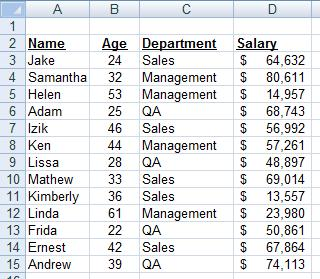Hide

# Sumif Function in Excel - an Easy Tutorial

Learn how to use the SUMIF function. Have a look at this free tutorial video, and if you need some more rehearsal take a look at the textual and illustrated tutorial below.

The sumif function enables you to sum up only some specific cells in a column, cells which confirm to some condition.
Have a look at the following worker's salary table:Let's for example, sum up the salary of all the workers who belong to the "Sales" department.

The sumif function consists of three parts separated by commas:
1. A range
2. A condition to apply to that range
3. The range of cells to sum up

In our example, the sumif will look literally something like this:
=sumif ( The Department column, is "Sales"? , The Salary column)

And the function will actually be:

=sumif(C3:C15,"Sales",D3:D15)

To sum up the salary of all workers of QA department, the function will be:

=sumif(C3:C15,"QA",D3:D15)

Another example:
Let's sum up the salary of all workers that are older than 35.

The function in words:
=sumif ( The Age column, is older than 35, The salary column)

And the actual function will be:

=sumif(B3:B15,">35",D3:D15)

Have a look at the tutorial video above to see an animated illustration of this subject.